# Algorithmic reducibility

(diff) ← Older revision | Latest revision (diff) | Newer revision → (diff)

One of the basic concepts in the theory of algorithms (cf. Algorithms, theory of) and its applications. Its background was the fact that solvability (and unsolvability) of many algorithmic problems (cf. Algorithmic problem) is not established directly, but rather by reducing some algorithmic problem, already demonstrated to be unsolvable, to the problem in question, or by reducing the latter problem to some other one which has already been solved. Thus, the problem of homotopy of paths in a polyhedron is shown to be unsolvable by reducing the problem of equality of words in the corresponding fundamental group to this problem.

In what follows, the simplest examples of algorithmic reducibility of number-theoretical predicates and functions (i.e. those defined on natural numbers) will be presented: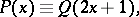whereand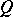are predicates. The problem of solving the predicate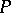, that is, of establishing the truth or falsehood offor different values of, is reduced to the problem of solving— or, "P is reduced to Q" , for short. One may also say that the set of truths of, i.e.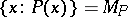, is reduced to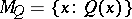.

Let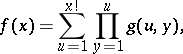where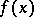and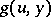are number-theoretic functions. The problem of computation of the functionis reduced to the computation of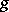or, for short,is reduced to.

The concept of algorithmic reducibility was given a more exact form by A.M. Turing: If, roughly speaking, some Turing machine transforms a sequence of encoded values of the functioninto a sequence of encoded values of the function, thenis reduced to. S.C. Kleene  formulated the related concept of relative computability with the aid of recursive systems of equations. After arithmetization, each algorithmic problem is reduced to the problem of computing some number-theoretic function. Ifis reduced toaccording to Turing,, andis reduced to,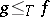, then one says thatandhave the same degree of unsolvability or that. The relation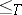is both reflexive and transitive. Thus, all functions (and sets of natural numbers or their characteristic predicates) are subdivided into equivalence classes, known as Turing degrees or-degrees . Most algorithmic problems considered in logic and in mathematics are expressible as problems of membership in enumerable sets of constructive objects. In this context the study of enumerable sets was initiated in the 1940s by E.L. Post ; he introduced certain special kinds of algorithmic reducibility, in addition to Turing's kind, and formulated the problem of reducibility as follows: Can various enumerable unsolvable sets be reduced (according to Turing) to each other? It was subsequently established that the enumerable sets form an infinite, very rich system of-degrees. This was supplemented by discovering the so-called priority method, which is extensively employed in the theory of algorithms.

Subsequently, degrees of unsolvability found applications in other branches of mathematics as well. For instance, for all-degreesandof enumerable sets, if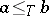, then there exists a finitely-determined group in which the problem of equality of words has degree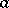, while the conjugation problem has degree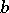. There is a close connection between degrees (concerning the different kinds of algorithmic reducibility) of enumerable sets and the rate of growth of the complexity of initial fragments of enumerable sets. A special kind of reducibility — polynomial reducibility or p-reducibility (reducibility with some limitation imposed on time) — is employed in proving the universal character of combinatorial optimization problems from various branches of mathematics ; for more details on this subject see . The methods of measure theory , and the forcing method  have in turn been used in the study of degrees.